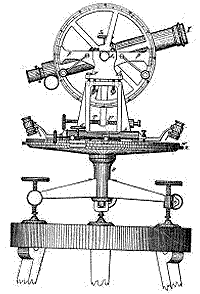Site Map Math Index Glossary Timeline Questions & Answers Lesson Plans #M-8. Sines and Cosines

# (M-7) Trigonometry--What it is good for?The basic problem of trigonometry runs somewhat like this: You stand next to a wide river and need to know the distance across it--say to a tree on the other shore, marked on the drawing here by the letter C (for simplicity, let's ignore the 3rd dimension). How can this be done without actually crossing the river? The usual prescription is as follows. Stick two poles in the ground at points A and B, and with a tape measure or surveyor's chain measure the distance c between them ("the baseline").An old surveyor's tele-  scope (theodolite).
Then remove the pole at A and replace it with a surveyor's telescope like the one shown here ("theodolite"), having a plate divided into 360 degrees, marking the direction ("azimuth") in which the telescope is pointing. Sighting the telescope, first at the tree and then at pole B, you measure the angle A of the triangle ABC, equal to the difference between the numbers you have read from the azimuth plate.. Replace the pole, take your scope to point B and measure the angle B in the same way.

The length c of the baseline, and the two angles A and B, contain all there is to know about the triangle ABC--enough, for instance, to construct a triangle of the same size and shape on some convenient open field. Trigonometry (trigon = triangle) was originally the art of deriving the missing information by pure calculation. Given enough information to define a triangle, trigonometry lets you calculate its remaining dimensions and angles.